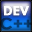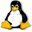Home Latest News Archive Tutorials C++ 2 3 4 Win32 Java OpenGL GLUT OpenGL ES 2 3 4 DirectX VB.NET HTML Photoshop 3dsmax Forums Projects Modelling Links About Us Search Recent Tutorials C / C++ Win32 OpenGL OpenGL ES GLUT### Introduction

When creating classes, you may want to take certain actions such as adding and subtracting two objects. As far as you know, you could achieve this by having a method called add or subtract. This could be made simpler by using specific operators such as +, -, +=, ++, /=, !, <<, etc.

Each operator can be used for anything eg. you could use the ! operator to give you the dot product of two vectors if you have a vector class.

An operator can be overloaded as follows by using the operator keyword :

return_type operator#(parameters);

where # is the operator.

Contents of main.cpp :

```#include <iostream>
#include <stdlib.h>

using namespace std;
```

This tutorial will make use of a money class. This allows money to be separated into dollars and cents. The constructors created are done so as per normal.

```class Money
{
public :

Money() {}
Money(int d, int c) :
dollars(d),
cents(c) {}
```

Overloading operators takes a little bit of thought, so we'll discuss each one in this tutorial separately.

Our first overloaded operator is the addition(+) operator. We do not want to return void as we may want to do something with the answer eg. a = b + c;

The method must take one parameter, being the right-hand side of the operation eg. a + b will call a's overloaded operator and b will be the parameter passed.

We pass the parameter by reference to avoid the unnecessary act of the parameter being copied. A const keyword is used as the method does not change any of the objects variables.

```	Money operator+(const Money &rhs) const;
```

Our next overloaded operator is the += operator. This is created in the exact same way as above except for 2 differences.

The one difference is that there is no const at the end of the declaration. This is because the variables are changed within the class.

The other difference is that the method returns a reference. This is because you want to return the actual object and not a copy of one. This is necessary if you want to use the resulting value eg. (a += b) += c. You may or may not want this functionality.

```	Money &operator+=(const Money &rhs);
```

Our next operator is the prefix addition(++) operator eg. ++c. Once again we return a reference for the same reason as above.

```	Money &operator++();
```

If we want to indicate the postfix instead of the prefix operator, all we need to do is use an int as a parameter. This identifies the postfix operator eg. c++. We have also added a const keyword before the rest of the declaration. This will be explained in greater detail below.

```	const Money operator++(int);
```

The rest of the class is created using techniques previously explained.

```	void printValue();

private :
// Getters and Setters
//---------------------
int getDollars() const
{ return dollars; }
void setDollars(int d)
{ dollars = d; }
int getCents() const
{ return cents; }
void setCents(int c)
{ cents = c; }

private :

int dollars;
int cents;
};
```

The method below simply output the money variable in the format "dollars.cents".

```void Money::printValue()
{
cout << dollars;
cout << ".";

if (!cents)
{
cout << "00";
}
else
{
if (cents < 10)
cout << "0";

cout << cents;
}

cout << endl;
}
```

The body of the overloaded + operator is given below.

```Money Money::operator+(const Money &rhs) const
{
```

We create a temporary object as we do not want to return the actual object.

```	Money temp;
```

Next step is to add the values of each object. Once this is done, a small calculation is used to determine if any extra dollars have to be added for every 100 cents.

```	temp.setDollars(this->dollars + rhs.getDollars());
temp.setCents(this->cents + rhs.getCents());

int incdollar = temp.getCents() / 100;
temp.setCents(temp.getCents() % 100);
temp.setDollars(temp.getDollars() + incdollar);
```

The temporary object is returned. Notice we do not need a copy constructor as there is no dynamic memory being used.

```	return temp;
}
```

The += operator uses the + operator above. Notice how we use the this pointer. We do not need to overload the = operator as there is no dynamic memory.

```Money &Money::operator+=(const Money &rhs)
{
*this = *this + rhs;
```

The actual object is returned by dereferencing the this pointer. You can now see more clearly why the this pointer is useful.

```	return *this;
```

The prefix operator is shown below. We increase the value and then return the new value(this).

```Money &Money::operator++()
{
this->dollars += 1;

return *this;
}
```

The postfix operator works in a similar manner except that a copy of the object is made before the increment. This is done as we are going to return the original value, thus giving the impression that the value is only increased after the line has been executed.

```const Money Money::operator++(int)
{
Money temp = *this;

this->dollars += 1;

return temp;
}
```

Below we give examples of how each operator can be used.

```int main()
{
Money a(50, 65);
Money b(32, 42);
Money c(0, 3);
```

The first example below show how the + operator returns another Money object.

The second example shows how the returned Money object can be used to continue adding extra Money objects.

```	// Addition
(a + b).printValue();
(a + b + c).printValue();
```

We can use the = operator normally as there is no dynamic memory.

```	Money d = c;
d.printValue();
```

Examples of how the increase operator can be used is shown below.

```	// Increase
cout << "Increment" << endl;
(c += c).printValue();
(c += c + a).printValue();
c.printValue();
a.printValue();
```

Examples of the postfix and prefix operators are given below. Notice how in the first example, the new value of c is used.
In the second example, the original value of d is used before the increment is made.

```	// Postfix & Prefix
cout << "Postfix & Prefix" << endl;
(++c + d).printValue();
(d++ + a).printValue();
d.printValue();
```

The code segment below shows how the const keyword in the postfix operator is useful. Notice that since we didn't place a const keyword at the beginning of the prefix operator, you can place many ++ operators before the variable. You may not want this. This is what the const keyword is used for. You can only place one ++ after the variable. This all depends on how you want to manipulate your objects.

```
++++++c;
//c++++++; // Error

system("pause");

return 0;
}```

Congratulations. A lot has been covered in this tutorial. Operator Overloading is extremely valuable when creating classes and it can save you a great amount of time while making your code easier to understand.

Please let me know of any comments you may have : Contact Me

 Source Files :